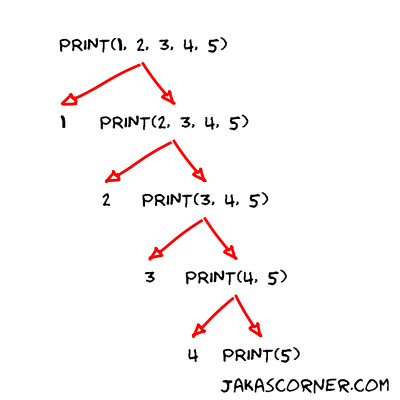The constructor of the `std::thread` is able to accept different number of arguments. It accepts a function and additionally all the arguments for this function. If the function `f` has n arguments, then the constructor looks like

If we would like to write concurrent code, we need to know how to handle functions (constructors) with variadic number of arguments. We need to know how to write such functions and we also need to know how to pass the arguments around. Let’s see how this is done.

## Functions with variadic number of arguments

We would like to write a function which prints all of its arguments. The function should be able to handle any number of arguments. With the C++11 standard, this is not so hard to do.

First function is simple. It receives a value of arbitrary type and then prints the value.

Second function is more interesting. It receives two arguments `first` and `args`. The `first` argument of type `T` represents the first argument of the function. The `args` argument of the type `Args...` represents all of the other arguments. The function prints the first argument and calls the function `print` with all the other arguments.

The `main` function contains some examples.

Running the program gives us the following output.

Let’s examine the `main()` function.

The `print(1)` calls the `print(T value)`. It prints `1` and then returns.

The `print(1, 2, 3, 4, 5)` calls the ```print(T first, Args... arg)``` function. The `first` then equals to `1` and the `args...` equals to ```2, 3, 4, 5```. The function prints `1` and calls `print(2, 3, 4, 5)`. The procedure continues until `print(5)` is called. After this call the `print(1, 2, 3, 4, 5)` returns and the main function proceeds with the next line.

You can visualize the `print(1, 2, 3, 4, 5)` like this:The `print("one", 2, "three", 4, "five")` demonstrates that we can also pass arguments of different types.

Programming the functions with variadic number of arguments feels like programming the recursive functions. Basically this is what we did: If the number of arguments is equal to one, we print the argument. If the number of arguments is greater than 1, we print the first argument and invoke `print` with the other arguments.

Technically, it is not a recursion, because every time different compiler generated function is called. But conceptually, I would call it a recursion.

The code does not look very clean. We recognize some repeating patterns. The main function calls `print` and then prints a new line. Our objective is to write such function which will print arbitrary number of arguments followed by a new line.

## Forwarding variadic number of arguments

Let’s write a function `println` which will forward the arguments to `print` and then it will additionally print a new line. The `println` should also accept variadic number of arguments.

The `Args&&...` and `std::forward<Args>...` are necessary for perfect forwarding of the arguments. Roughly speaking, the code ensures the following behavior: If the arguments support the move semantics they will be moved. If the arguments does not support the move semantics they will be passed by reference. For additional details about forwarding and move semantics I recommend the series of articles by Thomas Becker.

The main function now simplifies to

and it produces the same output as before.

## Summary

We learned how to handle a variadic number of arguments. This is specially important in concurrent programming, because we might want to write a code which could handle arbitrary number of arguments.